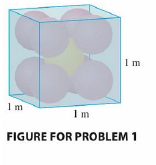Chapter 12, Problem 1P

Chapter
Section
Textbook Problem

# Each edge of a cubical box has length 1 m. The box contains nine spherical balls with the same radius r. The center of one ball is at the center of the cube and it touches the other eight balls. Each of the other eight balls touches three sides of the box. Thus the balls are tightly packed in the box (see the figure). Find r. (If you have trouble with this problem, read about the problem-solving strategy entitled Use Analogy on page 71.)To determine

To find: The radius of spherical ball.

Explanation

Given data:

The length of cube as 1 m.

The square and circle are analogous to cube and sphere in two-dimensional plane respectively.

Re-draw the analogous cross section diagram across diagonal of Figure for problem 1 in two-dimensional plane as shown in Figure 1.

Write the expression for diagonal of cube (d) .

d=3a (1)

Here,

a is length of square.

Substitute 1 m for a in equation (1),

d=3(1m)=3m

Write the expression for diagonal from Figure 1.

d=x+r+2r+r+x

d=4r+2x (2)

Here,

r is radius of sphere, and

x is diagonal of small square.

Substitute x for d and r for a in equation (1),

x=3r

Substitute 3 for d and 3r for x in equation (2),

3=4r+23r(4+23)r=3r=34+23

Multiply and divided by term 423 on right hand side of expression

### Still sussing out bartleby?

Check out a sample textbook solution.

See a sample solution

#### The Solution to Your Study Problems

Bartleby provides explanations to thousands of textbook problems written by our experts, many with advanced degrees!

Get Started

#### Using for |x| < 1 and differentiation, find a power series for .

Study Guide for Stewart's Single Variable Calculus: Early Transcendentals, 8th

#### Define the concept of internal validity and a threat to internal validity.

Research Methods for the Behavioral Sciences (MindTap Course List)

#### Self Check Graph: 3x2y=6.

College Algebra (MindTap Course List)